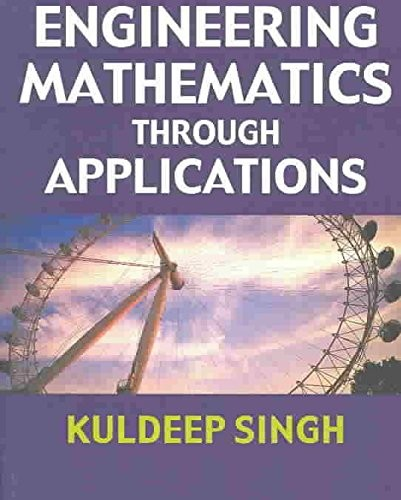# Engineering Mathematics Through Applications by Kuldeep Singh

In Stock
20,99 \$
inc. GST
Designed for engineering undergraduates with a low-level background in mathematics, this text teaches mathematics through an applications context drawing on a wide range of disciplines.
Only 1 left

## Engineering Mathematics Through Applications Summary

### Engineering Mathematics Through Applications by Kuldeep Singh

"Engineering Mathematics Through Applications" is a new textbook for all students on first-year engineering and pre-degree courses. It teaches mathematics in a step-by-step fashion, putting the mathematics into its engineering context at every stage. A comprehensive first-year course. Hundreds of examples and exercises, the majority set in an applied engineering context so that the students immediately see the purpose of what they are learning. Introductory chapter revises indices, fractions, decimals, percentages and ratios. Fully worked solutions to every problem on the companion website at www palgrave.com/science/engineering/singh Includes calculator and mathematical software examples and exercises. Student-friendly style encouraging active participation.

### Why buy from World of BooksOur excellent value books literally don't cost the earthFree delivery in AustraliaEvery used book bought is one saved from landfill

## Engineering Mathematics Through Applications Reviews

'The unique quality of this book is the wealth of examples applying the mathematical techniques taught here. These examples span mechanics, aerodynamics, electronics, engineering, fluid dynamics and other areas of applied mathematics. These are not just the usual examples involving differential equations and equations of motion, but real and thoughtful applications that will be relevant to the student.' - Jill Russell, Open University 'If you teach a first year mathematics module to a diverse engineering group, this book should be at the top of your list for consideration as a core text. It aims to encourage their [the students] learning through setting the mathematics within the context of engineering examples. With its very readable text it is suitable for both self-study and as support for a taught module. The book covers the requirements of most first year engineering mathematics modules with a fairly gentle reminder of arithmetic and algebra by way of introduction. Examples are drawn from such diverse subjects as electricity, control theory, heat flow, structures, fluid mechanics, signal processing, thermodynamics etc with the largest group being from mechanics. The examples are simple enough to be understood by most engineering students but sufficiently specific to allow students to see that mathematics is relevant to their own engineering discipline. I liked the presentation of the text - the questions posed to the reader, the full labelling in diagrams and the indication to students of the discipline of the examples. Helpful extra information is occasionally provided in subscript format to indicate the method used to move from one line to the next.' - Dr Ian Taylor, Faculty of Engineering at The University of Ulster, Engineering Subject Centre 'The book starts with the basics of mathematics making it suitable for those with little background in mathematics. This also makes it appealing to a wide variety of readers with different mathematical backgrounds. The book is a good size covering all the essential topics to adequate depth. It covers topics similar to other books targeted at the same audience; however it differs in that it does not assume readers to have a mathematical background. The book's approach of using examples is effective. Its use of examples is motivating. The engineering example generates interest by illustrating the importance and relevance of mathematics in engineering. A definite plus is the provision of online interactive questions, which enable users to test their understanding. This extra support makes it an ideal text for self-study and distance learning.' - Dr Lawrence Chirwa, School of Electrical and Mechanical Engineering at The University of Ulster, Engineering Subject Centre 'This is a book that is designed to cover the basics thoroughly and then move on. As a reminder of useful techniques, the book is definitely valuable. What is most refreshing is that it explains everything that you need to know in order to cover the basics of a given subject. The fact that there is almost no assumed knowledge is reassuring, and takes some of the mental strain away from getting your head around eigenvectors again... Essentially, if you want to remind yourself how to do those things that you once thought were straightforward, this is the book for you. A very useful book to have on your shelf.' - Edward Hoare, The Institution of Structural Engineers

KULDEEP SINGH is Senior Lecturer in the Department of Mathematics at the University of Hertfordshire. He teaches mathematics to a wide range of engineering and science students.

Note to the Student Preface Introduction: Arithmetic for Engineers Engineering Formulae Visualizing Engineering Formulae Functions on Engineering Trigonometry and Waveforms Logarithmic, Exponential and Hyperbolic Functions Differentiation Engineering Applications of Differentiation Integration Engineering Applications of Integration Complex Numbers Matrices Vectors First Order Differential Equations Second Order Linear Differential Equations Partial Differentiation Probability and Statistics Solutions Appendix: Standard Normal Distribution Table Index

GOR002658700
Engineering Mathematics Through Applications by Kuldeep Singh
Kuldeep Singh
Used - Very Good
Paperback
Palgrave Macmillan
2003-02-19
840
0333922247
9780333922248
N/A
Book picture is for illustrative purposes only, actual binding, cover or edition may vary.
This is a used book - there is no escaping the fact it has been read by someone else and it will show signs of wear and previous use. Overall we expect it to be in very good condition, but if you are not entirely satisfied please get in touch with us.